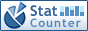Home PC Games Linux Windows Database Network Programming Server Mobile
 Home \ Programming \ Understanding the type in C ++ bitset - To install and deploy Java applications under CentOS 6.5 (Linux) - Compare Oracle MySQL (ICP) index condition pushdown is described (Database) - CentOS 6.5 using Virtualenv under development environment to build Python3 (Linux) - How to interactively create a Docker container (Server) - Install the system cleaning software under Linux: BleachBit 1.4 (Linux) - C ++ Supplements --new delete overload (Programming) - ImageMagick Tutorial: How to cut images in Linux command line (Linux) - MySQL Error Code Complete (Database) - Ubuntu 15.04 and CentOS 7 to deploy .NET, Mono and DNX (Server) - CentOS 7 repair MBR and GRUB (Linux) - Linux platform to prevent hackers to share practical skills (Linux) - JavaScript closures and the scope chain (Programming) - Use top to monitor the remote server (Server) - Android development environment to build under Fedora 13 (Linux) - MongoDB3.0.6 wiredtiger MMAPv1 (Database) - The three-way division of the sorting algorithm Quicksort (Programming) - JavaScript notes --Objects (Programming) - Linux System Getting Started Learning: Statistical process a number of threads in Linux (Linux) - Bootable ISO image using GRUB (Linux) - Construction Spark source and application development environment (Server) Understanding the type in C ++ bitset Add Date : 2017-08-31 Understanding the type of standard library bitset Bit is used to save a group of items or conditions yes / no (1 or 0) A simple method of information, then the bits set is an ordered set of bits. bitset class C ++ standard library provides very effective in our program simplifies the process for the position set. Defined bitset object We want to use bitset, you first need to include the header file bitset. And vector objects is not the same type of distinction bitset object only in its length but not in its type. In the definition of bitset time, to clear bitset contains the number of bits required to give its length value in angle brackets. Length value must be defined as shaping literals or integer constant values initialized const object is used. 1 const int maxn = 16; 2 bitset <32> bitvec2; 3 bitset bitvec (1); bitset to the beginning of the bit string 0 is the low order bit, bit string to the end of the 31 high-order bits. Bitset object initialization 1. unsigned value is initialized When using unsigned long value as the initial value of the bitset object, the value will be converted to a binary bit pattern. The bitset object position set as a copy of this bit pattern. If the length is greater than bitset type unsigned long value of binary digits, the remaining high-order bit is set to 0; if bitset type unsigned long length is less than the value of binary digits, only the low-order bit unsigned values over order bit bitset type length will be discarded. 1 bitset <32> bitvec3 (0xffff); 2 cout << bitvec3 << endl; 3 for (int i = 0; i 4 cout << bitvec3 [i] << ""; 5 cout << endl; 2. The object is initialized with a string bitset objects When the object is initialized with a string bitset objects, string objects directly represented as a bit pattern. Read bit set from a string target sequence is from right to left. 1 string str ( "11100"); 2 bitset <8> bitvec5 (str); 3 cout << bitvec5 << endl; 4 for (int i = 0; i 5 cout << bitvec5 [i] << ""; Note: It is the reverse conversion between string objects and bitset objects, string objects rightmost characters (ie the largest subscript characters) is used to initialize the bitset object's lower order bits (ie, subscript 0 bit). Access bitset object position And the same vector, bitset can also read and write an index position bits by the subscript operator, the same, you can also use the subscript operator to test the value of a given set of bits or a binary value of the bit. In fact, access bitset object bit is to get a for loop, the code above illustrations have mentioned here, which will not be described in detail. bitset manipulation functions bitset implementation manipulation functions #include < iostream > #include < cstdio > #include < cstdlib > #include < cstring > #include < cmath > #include < algorithm > #include < bitset > using namespace std; const int maxn = 16; int main () {     bitset <16> b2;     cout << "b2.any () =" << b2.any () << endl;     cout << "b2.none () =" << b2.none () << endl;     cout << "b2.size () =" << b2.size () << endl;     cout << "b2  =" << b2  << endl;     cout << "b2.test (4) =" << b2.test (4) << endl;     b2.set (); cout << endl;     cout << "b2.any () =" << b2.any () << endl;     cout << "b2.none () =" << b2.none () << endl;     cout << "b2.size () =" << b2.size () << endl;     cout << "b2  =" << b2  << endl;     cout << "b2.test (4) =" << b2.test (4) << endl;     b2.reset (); cout << endl;     cout << "b2.any () =" << b2.any () << endl;     cout << "b2.none () =" << b2.none () << endl;     cout << "b2.size () =" << b2.size () << endl;     cout << "b2  =" << b2  << endl;     cout << "b2.test (4) =" << b2.test (4) << endl;     b2.set (4); cout << endl;     cout << "b2  =" << b2  << endl;     cout << "b2.test (4) =" << b2.test (4) << endl;     return 0; } More:
 CopyRight 2002-2020 newfreesoft.com, All Rights Reserved.